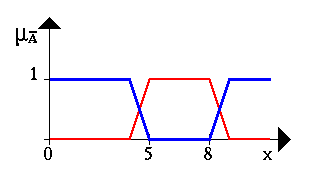# Operations on Fuzzy Sets

Now that we have an idea of what fuzzy sets are, we can introduce basic operations on fuzzy sets. Similar to the operations on crisp sets we also want to intersect, unify and negate fuzzy sets. In his very first paper about fuzzy sets, L. A. Zadeh suggested the minimum operator for the intersection and the maximum operator for the union of two fuzzy sets. It is easy to see that these operators coincide with the crisp unification, and intersection if we only consider the membership degrees 0 and 1.

In order to clarify this, we give a few examples. Let A be a fuzzy interval between 5 and 8 and B be a fuzzy number about 4. The corresponding figures are shown below.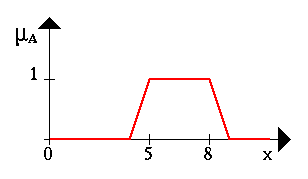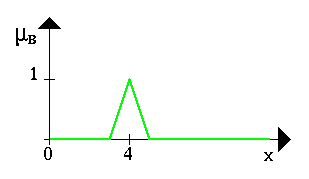The following figure shows the fuzzy set between 5 and 8 AND about 4 (notice the blue line).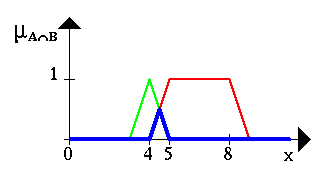The Fuzzy set between 5 and 8 OR about 4 is shown in the next figure (again, it is the blue line).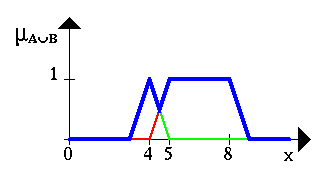This figure gives an example for a negation. The blue line is the NEGATION of the fuzzy set A.